# 1st PUC Maths Question Bank Chapter 4 Principle of Mathematical Induction

## Karnataka 1st PUC Maths Question Bank Chapter 4 Principle of Mathematical Induction

Question 1.
Using the principle of mathematical induction, prove that
$$\text { (1) } 1+2+3+\cdots+n=\frac{n(n+1)}{2}$$, for all n∈N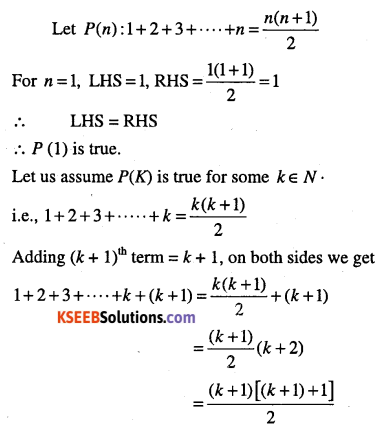which is P(k +1)
Thus, P(k)⇒ P(k +1).
Hence, by mathematical induction P(n) is true for all n∈NQuestion 2.
1 + 3 + 5 + 7 + …….. + (2n – 1) = n2, for all n∈N
Let P(n):1 + 3 + 5 + 7+……….. +(2n-1) = n2
For n = 1, LHS = 1, RHS = 12 = 1
∴ LHS = RHS
∴ F(1) is true.
Let upwards assume P(k) is true for some k∈N
i.e., 1 + 3 + 5 + …. + (2K- 1) = kz
Adding (k + 1)th term = 2K + 1, on both sides, we get,
1 + 3 + 5 +……. +(2k -1)(2k + l) = k2 + 2k + 1
= (k +1)2
which is P(k +1)
Thus, P(k)⇒ P(k +1).
Hence, by mathematical induction P(n) is true for all n∈N

Question 3.
$$1^{2}+2^{2}+3^{2}+\ldots \ldots+n^{2}=\frac{n(n+1)(2 n+1)}{6}$$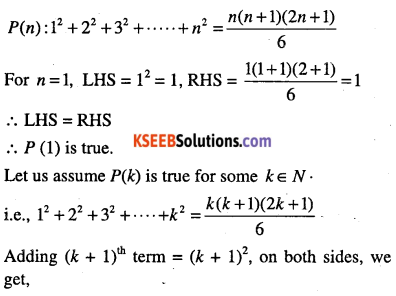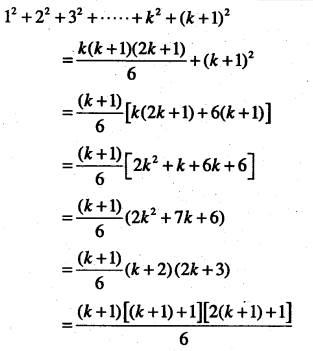which is P(k +1)
Thus, P(k) ⇒ P(k +1).
Hence, by mathematical induction P(n) is true for all n∈NQuestion 4.
$$1^{3}+2^{3}+3^{3}+\ldots \ldots+n^{3}=\left[\frac{n(n+1)}{2}\right]^{2}$$ for all n∈N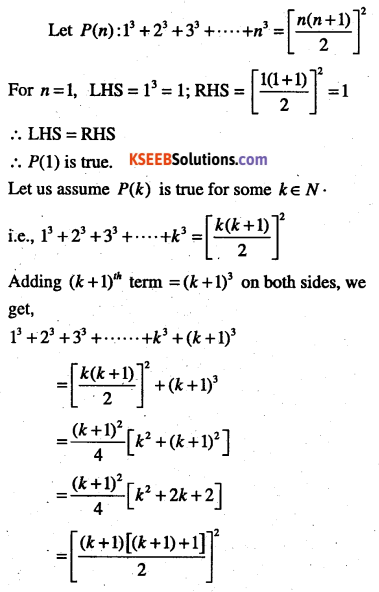which is P(k +1)
Thus, P(k) ⇒ P(k +1).
Hence, by mathematical induction P(n) is true for all n∈N

Question 5.
$$1 \cdot 2+2 \cdot 3+\dots+n(n+1)=\frac{n(n+1)(n+2)}{3}$$ for all n∈N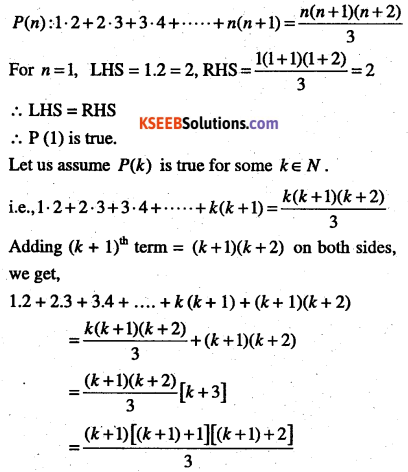which is P(k +1)
Thus, P(k) ⇒ P(k +1).
Hence, by mathematical induction P(n) is true for all n∈N

Question 6.
\begin{aligned} &1 \cdot 2 \cdot 3+2 \cdot 3 \cdot 4+\dots+n(n+1)(n+2)&=\frac{n(n+1)(n+2)(n+3)}{4}, \text { for all } n \in N \end{aligned}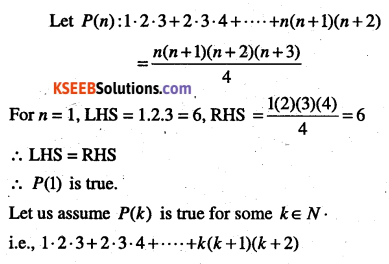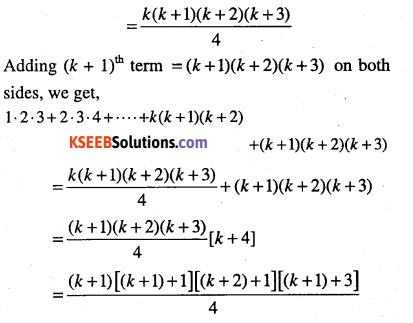which is P(k +1)
Thus, P(k) ⇒ P(k +1).
Hence, by mathematical induction P(n) is true for all n∈N

Question 7.
$$\cfrac{1}{1 \cdot 2}+\cfrac{1}{2 \cdot 3}+\cfrac{1}{3 \cdot 4}+\dots+\cfrac{1}{n(n+1)}=\cfrac{n}{n+1}$$ for all n∈N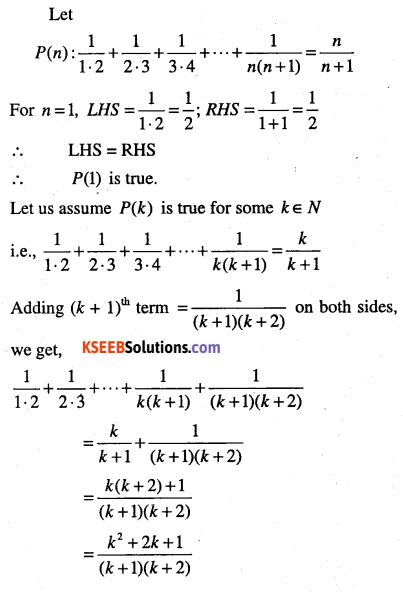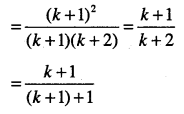which is P(k +1)
Thus, P(k) ⇒ P(k +1).
Hence, by mathematical induction P(n) is true for all n∈N

Question 8.
\begin{aligned} &\cfrac{1}{1 \cdot 2 \cdot 3}+\cfrac{1}{2 \cdot 3 \cdot 4}+\cfrac{1}{3 \cdot 4 \cdot 5}+\dots+\cfrac{1}{n(n+1)(n+2)}&=\cfrac{n(n+3)}{4(n+1)(n+2)}, \text { for all } n \in N \end{aligned}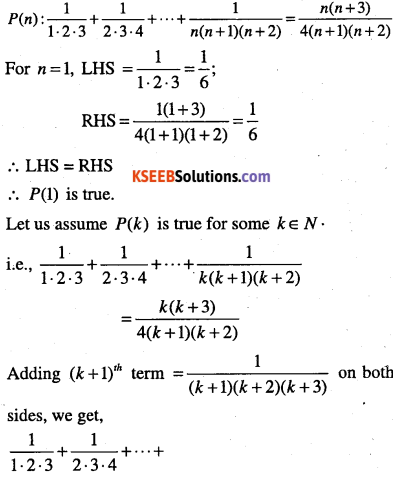which is P(k +1)
Thus, P(k) ⇒ P(k +1).
Hence, by mathematical induction P(n) is true for all n∈NQuestion 9.
\begin{aligned}&\frac{1}{2 \cdot 5}+\frac{1}{5 \cdot 8}+\frac{1}{8 \cdot 11}+\cdots \cdot+\\ &\frac{1}{(3 n-1)(3 n+2)}=\frac{n}{6 n+4} \end{aligned}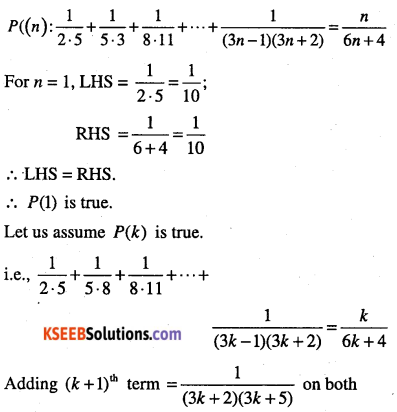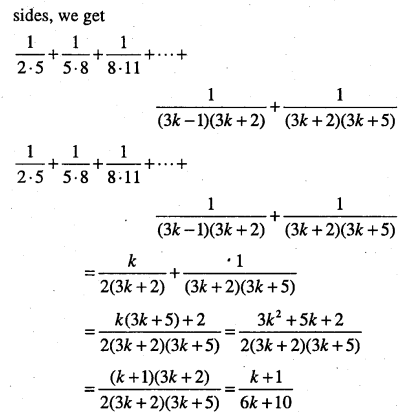which is P(k +1)
Thus, P(k) ⇒ P(k +1).
Hence, by mathematical induction P(n) is true for all n∈N

Question 10.
\begin{aligned} &\cfrac{1}{1 \cdot 4}+\cfrac{1}{4 \cdot 7}+\cfrac{1}{7 \cdot 10}+\cdots+\\ &\cfrac{1}{(3 n-2)(3 n+1)}=\cfrac{n}{3 n+1} \end{aligned}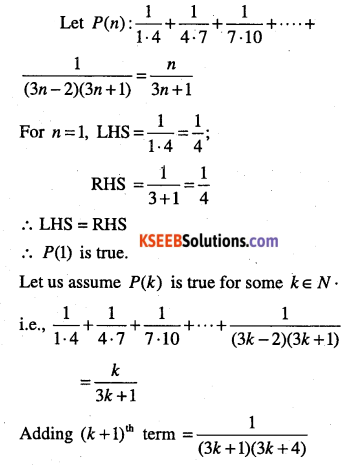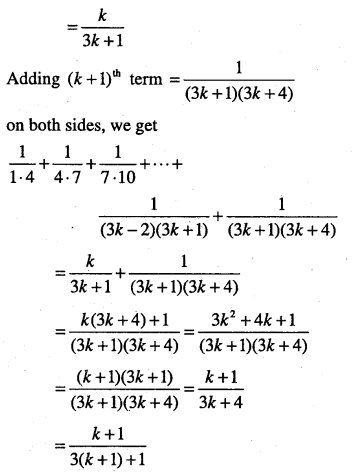which is P(k +1)
Thus, P(k) ⇒ P(k +1).
Hence, by mathematical induction P(n) is true for all n∈N

Question 11.
\begin{aligned} &\cfrac{1}{3 \cdot 5}+\cfrac{1}{5 \cdot 7}+\cfrac{1}{7 \cdot 9}+\cdots+\\ &\cfrac{1}{(2 n+1)(2 n+3)}=\cfrac{n}{3(2 n+3)} \end{aligned}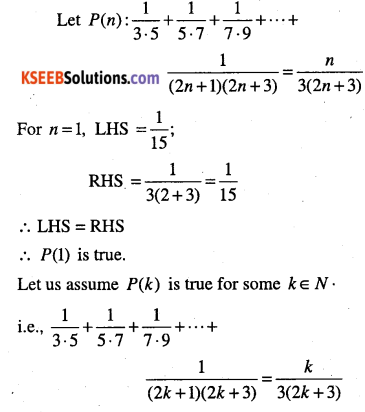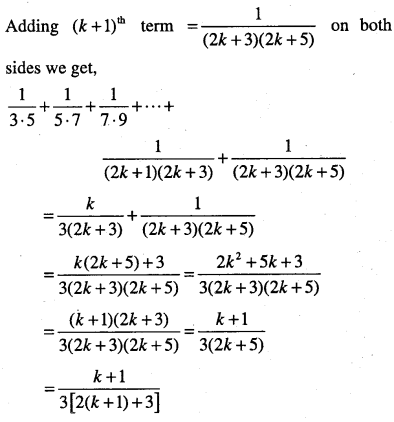which is P(k +1)
Thus, P(k) ⇒ P(k +1).
Hence, by mathematical induction P(n) is true for all n∈N

Question 12.
\begin{aligned} &1+\cfrac{1}{1+2}+\cfrac{1}{1+2+3}+\dots+\\ &\cfrac{1}{1+2+3+\dots+n}=\cfrac{2 n}{n+1} \end{aligned}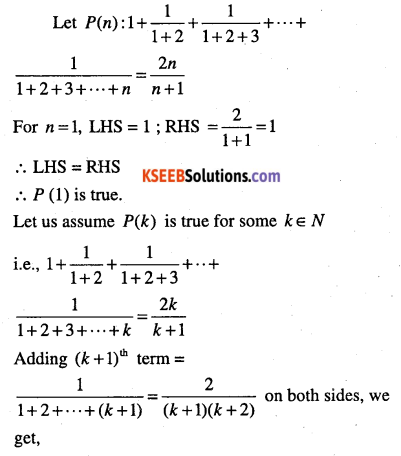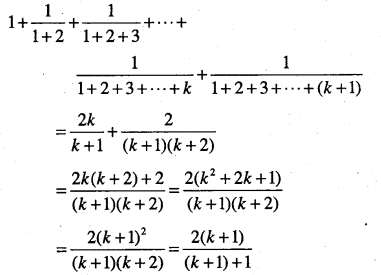which is P(k +1)
Thus, P(k) ⇒ P(k +1).
Hence, by mathematical induction P(n) is true for all n∈NQuestion 13.
\begin{aligned}&1 \cdot 3+3 \cdot 5+5 \cdot 7+\dots+(2 n-1)(2 n+1)&=\cfrac{n\left(4 n^{2}+6 n-1\right)}{3}, \text { for all } n \in N \end{aligned}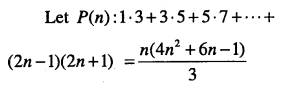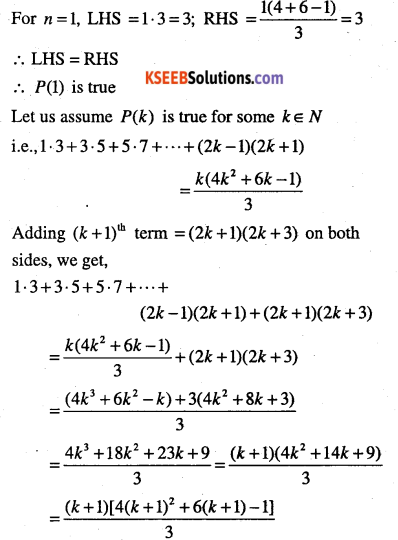which is P(k +1)
Thus, P(k) ⇒ P(k +1).
Hence, by mathematical induction P(n) is true for all n∈N

Question 14.
$$1+3+3^{2}+\cdots+3^{n-1}=\cfrac{3^{n}-1}{2}$$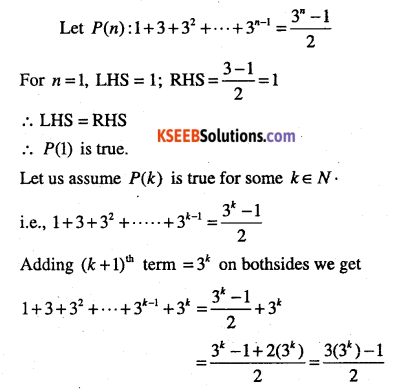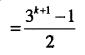which is P(k +1)
Thus, P(k) ⇒ P(k +1).
Hence, by mathematical induction P(n) is true for all n∈N

Question 15.
$$\cfrac{1}{2}+\cfrac{1}{4}+\cfrac{1}{8}+\dots+\cfrac{1}{2^{n}}=1-\cfrac{1}{2^{n}}$$which is P(k +1)
Thus, P(k) ⇒ P(k +1).
Hence, by mathematical induction P(n) is true for all n∈N

Question 16.
$$a+a r+a r^{2}+\cdots+a r^{n-1}=\cfrac{a\left(r^{n}-1\right)}{r-1}$$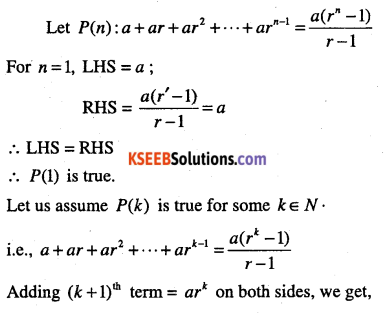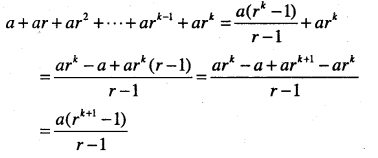which is P(k +1)
Thus, P(k) ⇒ P(k +1).
Hence, by mathematical induction P(n) is true for all n∈N

Question 17.
$$1 \cdot 2+2 \cdot 2^{2}+3 \cdot 2^{3}+\cdots+n 2^{n}=(n-1) 2^{n+1}+2$$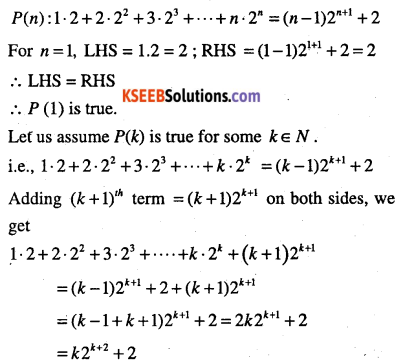which is P(k +1)
Thus, P(k) ⇒ P(k +1).
Hence, by mathematical induction P(n) is true for all n∈N

Question 18.
\begin{aligned} &1 \cdot 3+2 \cdot 3^{2}+3 \cdot 3^{2}+\cdots+n \cdot 3^{n}&=\cfrac{(2 n-1) 3^{n+1}+3}{4}, \text { for all } n \in N \end{aligned}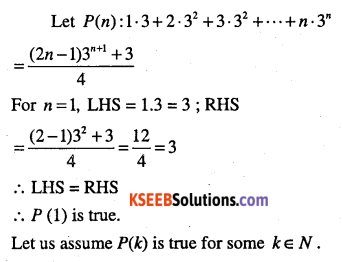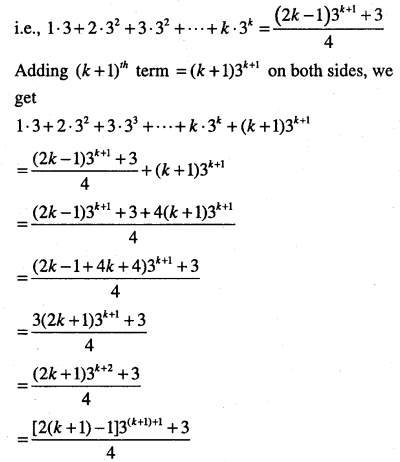which is P(k +1)
Thus, P(k) ⇒ P(k +1).
Hence, by mathematical induction P(n) is true for all n∈NQuestion 19.
$$\left(1+\frac{1}{1}\right)\left(1+\frac{1}{2}\right)\left(1+\frac{1}{3}\right) \cdots\left(1+\frac{1}{n}\right)=n+1$$ for all n∈N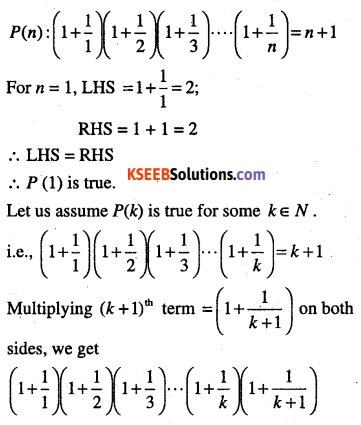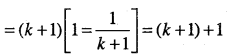which is P(k +1)
Thus, P(k) ⇒ P(k +1).
Hence, by mathematical induction P(n) is true for all n∈N

Question 20.
$$\left(1+\cfrac{3}{1}\right)\left(1+\cfrac{5}{4}\right)\left(1+\cfrac{7}{9}\right) \cdots\left(1+\cfrac{2 n+1}{n^{2}}\right)=(n+1)^{2}$$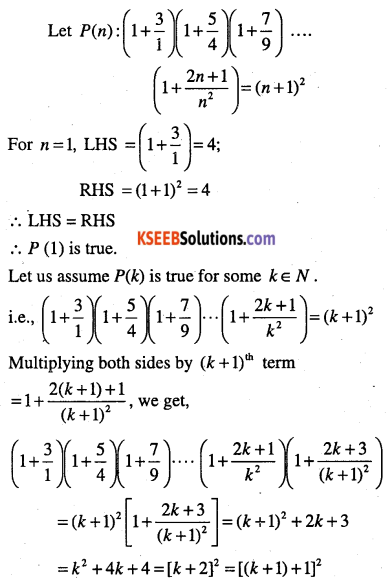which is P(k +1)
Thus, P(k) ⇒ P(k +1).
Hence, by mathematical induction P(n) is true for all n∈N

Question 21.
$$1^{2}+3^{2}+5^{2}+\cdots \cdot \cdot+(2 n-1)^{2}=\cfrac{n(2 n-1)(2 n+1)}{3}$$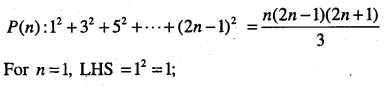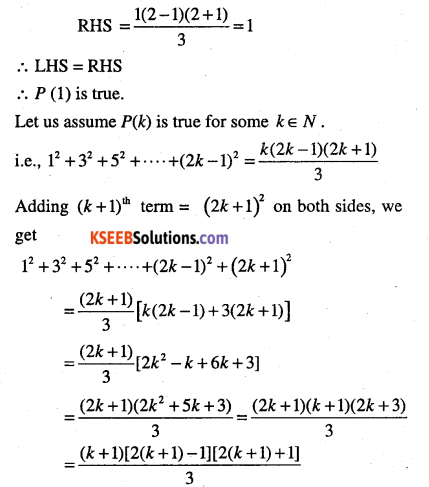which is P(k +1)
Thus, P(k) ⇒ P(k +1).
Hence, by mathematical induction P(n) is true for all n∈N

Question 22.
Prove that 2n> n for all positives integers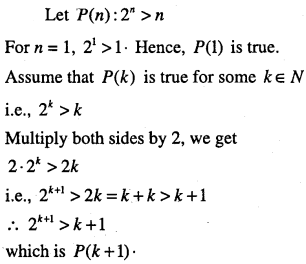Thus, P(k) ⇒ P(k +1).
Hence, by mathematical induction P(n) is true for all n∈N

Question 23.
$$1+2+3 \cdots+n<\frac{1}{8}(2 n+1)^{2}$$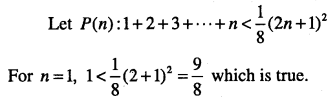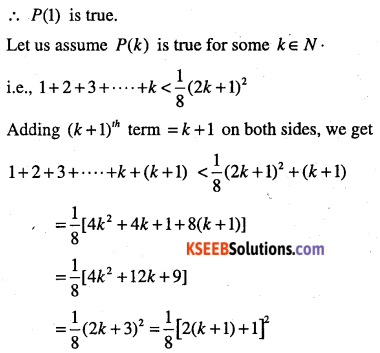which is P(k +1)
Thus, P(k) ⇒ P(k +1).
Hence, by mathematical induction P(n) is true for all n∈NQuestion 24.
$$1^{2}+2^{2}+3^{2}+\cdots+n^{2}>\frac{n^{3}}{3}, n \in N$$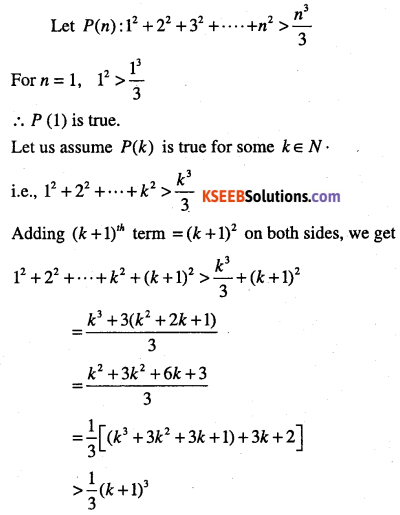which is P(k +1)
Thus, P(k) ⇒ P(k +1).
Hence, by mathematical induction P(n) is true for all n∈N

Question 25.
(2n + 7) < (n + 3)2
Let P(n): (2n + 7) < (n + 3)2
For = 1, (2 + 7)<(1+ 3)2 ⇒9<16 is true.
∴ P(1) is true.
Let us assume P(k) is true for some k∈ N
i.e., (2k+ 1) < (k + 3)2
Consider 2 (k + 1) + 7 = 2k + 2 + 7
= (2k + 7) + 2
< (k: + 3)2 + 2 = k2 + 6k + 9 + 2
<(k + 4)2
∴ P(k +1) is true.
Hence, by mathematical induction, P(n) is true for all n∈N

Question 26.
72n – 32n is divisible by 4.
Let P(n): 7n – 3n is divisible by 4.
For n = 1, P(1): 71 – 31 = 4 which is divisible by 4.
∴ P(1) is true.
Let us assume P(k) is true for some k∈N
i.e., 72n – 32n is divisible by 4.
Thus, P(k) ⇒ P(k +1).
Hence, by mathematical induction P(n) is true for all n∈N

Question 27.
x2n – y2n is divisible by x+y.
Let P(n): x2n – y2n is divisible by x + y
For n = 1, P(1): x2 – y2 is divisible by x + y, which is true.
∴ P(1) is true.
Let us assume P(k) is true for some k∈ N
i.e., x2k – y2k is divisible by x + y
Let x2k – y2k = (x + y)d, where d∈N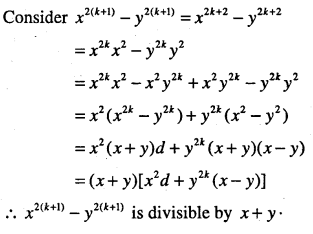which is P(k +1)
Thus, P(k) ⇒ P(k +1).
Hence, by mathematical induction P(n) is true for all n∈N

Question 28.
102n-1 +1 is divisible by
Let P(n): 102n-1 +1, is divisible by 11.
For n = 1, P(1):102-1 +1, is divisible by 11, which is true.
P(1) is true.
Let us assume P(k) is true for some k∈N.
i.e., 102n-1+1 is divisible by 11.
⇒102-1 +1 = 1 W, d∈N.
Consider 102(k+1)-1+1 = 102k+1 +1
= 102k-1 102+1 = (11d-1)102+1
= 11d(102)-100 + 1 = 11(100d)-99
= 11(100d-9)
∴ 102(k+1)-1 +1 is divisible by 11.
Thus, P(k) ⇒ P(k +1)
Hence, by mathematical induction, P(n) is true for all n∈N

Question 29.
32n+2 -8n-9 is divisible by 8.
Let P(n): 32n+2 -8n-9 is divisible by 8.
For n = 1, P(1): 32+2 – 8(2) – 9 = 64 is divisible by 8, which is true.
P( 1) is true.
Let us assume P(k) is true for some k∈N
i.e., 32k+2 -8k -9 is divisible by 8.
Let 32k+2-8k-9 = 8d, d∈N ………………(1)
Consider 32(k+1)+2 – 8(k +1) – 9 = 32k+4 – 8k – 8 – 9
= 32k+2 -32-8(k-1) = (8d + 8k + 9)9-8k-17 using (1)
= (8d + 8k)9 + 81-8k-17
= 8(d + k)9 + 64-8k = 8[9(d + k) + 8-k]
which is divisible by 8.
Thus, P(k) ⇒ P(k +1)
Hence, by mathematical induction, P(n) is true for all n∈NQuestion 30.
2.7n + 3.5n – 5 is divisible by 24 for all n∈N
Let P(n): 2.7n + 3.5n – 5 is divisible by 24.
For n = 1; P(1): 2.7n + 3.5n – 5 = 24 is divisible by 24.              ‘
∴ P(1) is true.
Let us assume, P(k) is true for some k∈N
i.e P(n): 2.7k + 3.5k – 5 is divisible by 24.
Let 2.7k + 3.5k – 5=24d,where d∈N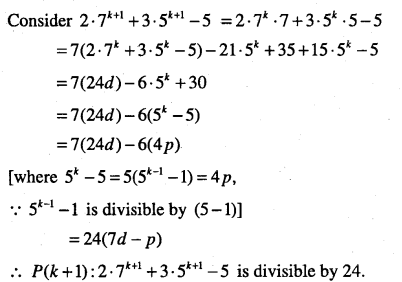Thus, P(k)⇒P(k+ l)
Hence, by mathematical induction, P(n) is true for all n∈N

Question 31.
41n -14n  is a multiple of 27.
Let P(n): 41n -14n is a multiple of 27.
For n = 1, P(1): 41′-14′ = 47 is a multiple of 27.
∴ P(1) is true.
Let us assume P(k) is true for some k∈N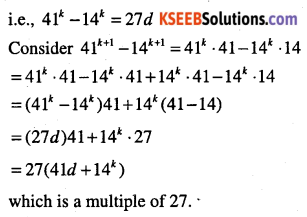Thus, P(k)⇒P(k+ 1)
Hence, by mathematical induction, P(n) is true for all n∈N

Question 32.
n(n +1)(n + 5) is a multiple of 3.
Let P(n): n(n + 1)(n + 5) is a multiple of 3.
For n = 1, P(1): 1(1 +1)(1 + 5) = 12 is a multiple of 3.
∴ P(1) is true.
Let us assume P(k) is true for some n∈N
i.e., P(k):k(k + 1)(k + 5) is a multiple of 3.
Let k(k + 1)(k + 5) = 3d
Consider (k + 1)(k + 2)(k + 6)
= (k + 2)(k + 1)(k + 5 + 1)
= k(k + 1)(k + 5 + 1) + 2(k + 1)(k + 5 + 1)
= k(k +1 )(k + 5) + k(k +1) + 2(k +1 )(k + 6)

Question 33.
$$(a b)^{n}=a^{n} b^{n}$$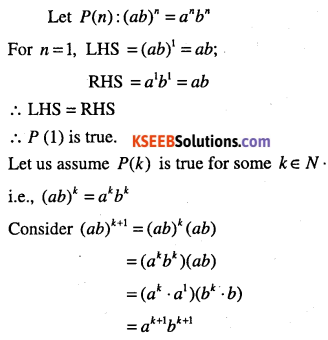Question 34.
(1 + x)n ≥ (1 + nx)for all natural number n, where x>-l.